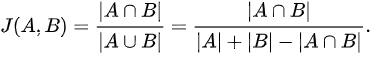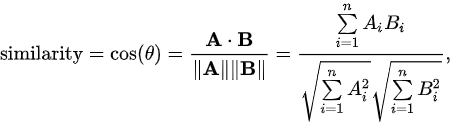Similarity Primitives¶

Overview¶

In graph theory, a similarity measure or similarity function is an real-valued function which indicates whether two vertices are similar to each other (from wikipedia). The API will provide the two commonly used similarity function which are Jaccard Similarity and Cosine Similarity.

Jaccard Similarity Algorithm¶

Jaccard similarity is defined as the size of the intersection divided by the size of the union of the sample sets:If both A and B are empty, the J(A, B) is defined as 1. Care must be taken if the union of A and B is zero or infinite. In that case, J(A, B) is not defined. Jaccard Similarity is widely used in object detection as a judegment of similarity between the detection rectangular and the ground truth.

Cosine Similarity Algorithm¶

Cosine similarity is a measure of similarity between two non-zero vectors of an inner product space. It is defined to equal the cosine of the angle between them, which is also the same as the inner product of the same vectors normalized to both have length 1. The result of cosine value is between 0 and 1. If the result is 1, it indiceate the two vector are exactly the same. The formula of cosine similarity is:Cosine Similarity is mainly used for measuring the distance between different text file. It is advantageous because even if the two similar documents are far apart by the Euclidean distance because of the size they could still have a smaller angle between them.